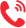更多 >>

学校：
地区：

华东交通大学
• 专业名称
• 所属学院
• 学制
• 学费(元)
• 地区
• 简章详情
• 报名
江西师范大学
• 专业名称
• 所属学院
• 学制
• 学费(元)
• 地区
• 简章详情
• 报名
西北师范大学

清华大学
• 专业名称
• 所属学院
• 学制
• 学费(元)
• 地区
• 简章详情
• 报名
同济大学
• 专业名称
• 所属学院
• 学制
• 学费(元)
• 地区
• 简章详情
• 报名
华东师范大学
• 专业名称
• 所属学院
• 学制
• 学费(元)
• 地区
• 简章详情
• 报名
浙江大学
• 专业名称
• 所属学院
• 学制
• 学费(元)
• 地区
• 简章详情
• 报名
四川大学
• 专业名称
• 所属学院
• 学制
• 学费(元)
• 地区
• 简章详情
• 报名
太原理工大学
• 专业名称
• 所属学院
• 学制
• 学费(元)
• 地区
• 简章详情
• 报名
东北师范大学
• 专业名称
• 所属学院
• 学制
• 学费(元)
• 地区
• 简章详情
• 报名
苏州大学
• 专业名称
• 所属学院
• 学制
• 学费(元)
• 地区
• 简章详情
• 报名
湖南师范大学
• 专业名称
• 所属学院
• 学制
• 学费(元)
• 地区
• 简章详情
• 报名
华南师范大学
• 专业名称
• 所属学院
• 学制
• 学费(元)
• 地区
• 简章详情
• 报名
中国矿业大学(北京)
• 专业名称
• 所属学院
• 学制
• 学费(元)
• 地区
• 简章详情
• 报名
中国地质大学(北京)
• 专业名称
• 所属学院
• 学制
• 学费(元)
• 地区
• 简章详情
• 报名
中北大学
• 专业名称
• 所属学院
• 学制
• 学费(元)
• 地区
• 简章详情
• 报名
沈阳师范大学
• 专业名称
• 所属学院
• 学制
• 学费(元)
• 地区
• 简章详情
• 报名
吉林师范大学
• 专业名称
• 所属学院
• 学制
• 学费(元)
• 地区
• 简章详情
• 报名
齐齐哈尔大学
• 专业名称
• 所属学院
• 学制
• 学费(元)
• 地区
• 简章详情
• 报名
安徽师范大学
• 专业名称
• 所属学院
• 学制
• 学费(元)
• 地区
• 简章详情
• 报名
集美大学
• 专业名称
• 所属学院
• 学制
• 学费(元)
• 地区
• 简章详情
• 报名
福建师范大学
• 专业名称
• 所属学院
• 学制
• 学费(元)
• 地区
• 简章详情
• 报名
华东交通大学
• 专业名称
• 所属学院
• 学制
• 学费(元)
• 地区
• 简章详情
• 报名
聊城大学
• 专业名称
• 所属学院
• 学制
• 学费(元)
• 地区
• 简章详情
• 报名
河南师范大学
• 专业名称
• 所属学院
• 学制
• 学费(元)
• 地区
• 简章详情
• 报名
湖南农业大学
• 专业名称
• 所属学院
• 学制
• 学费(元)
• 地区
• 简章详情
• 报名
广西师范大学
• 专业名称
• 所属学院
• 学制
• 学费(元)
• 地区
• 简章详情
• 报名
贵州师范大学
• 专业名称
• 所属学院
• 学制
• 学费(元)
• 地区
• 简章详情
• 报名
云南农业大学
• 专业名称
• 所属学院
• 学制
• 学费(元)
• 地区
• 简章详情
• 报名
西安石油大学
• 专业名称
• 所属学院
• 学制
• 学费(元)
• 地区
• 简章详情
• 报名
江汉大学
• 专业名称
• 所属学院
• 学制
• 学费(元)
• 地区
• 简章详情
• 报名
三峡大学
• 专业名称
• 所属学院
• 学制
• 学费(元)
• 地区
• 简章详情
• 报名
扬州大学
• 专业名称
• 所属学院
• 学制
• 学费(元)
• 地区
• 简章详情
• 报名
江西科技师范大学
• 专业名称
• 所属学院
• 学制
• 学费(元)
• 地区
• 简章详情
• 报名
宁波大学
• 专业名称
• 所属学院
• 学制
• 学费(元)
• 地区
• 简章详情
• 报名
河北科技师范学院
• 专业名称
• 所属学院
• 学制
• 学费(元)
• 地区
• 简章详情
• 报名

更多 >>

更多 >>

## 联系方式400-101-7010

报名咨询热线

400-101-7010